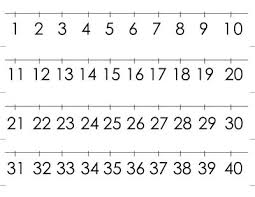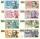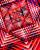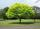# Divisible by four

Are 240 divisible by 4?

Result

x=

### Step-by-step explanation:Did you find an error or inaccuracy? Feel free to write us. Thank you!Tips to related online calculators
Do you want to perform natural numbers division - find the quotient and remainder?

## Related math problems and questions:

• Ornamental shrubsChildren committed to planting 240 ornamental shrubs. Their commitment, however, exceeded by 48 shrubs. Write the ratio of actually planted shrubs and commitment by lowest possible integers a/b.
• PegsFrom two sticks 240 cm and 210 cm long, it is necessary to cut the longest possible pegs for flowers so that no residues remain. How many pegs will it be?
• How manyHow many integers are greater than 547/3 and less than 931/4?
• RemaindersIt is given a set of numbers { 170; 244; 299; 333; 351; 391; 423; 644 }. Divide this numbers by number 66 and determine set of remainders. As result write sum of this remainders.
• Divisible by nineHow many three-digit natural numbers in total are divisible without a remainder by the number 9?
• Cash backAfter a trip 24 students have left more than 650 but less than 690 CZK (Kc). How much each student receives return when the amount is divisible by the number of pupils?
• What are 3What are the two digits which when inserted in the blank spaces will make 234 _ _ divisible by 8?
• By sixFrom the digits 1,2,3,4 we create the long integer number 123412341234. .. .. , which will have 962 digits. Is this number divisible by 6?
• ConcertOn a Concert were sold 150 tickets for CZK 360, 235 tickets for 240 CZK and 412 for 180 CZK. How much was the total revenues for tickets?
• AmanAman, Anna and Tina are participating in the school race. Anna completes a lap in 120 minutes, Aman completes a lap in 240 minutes and Tina completes a lap in 60 minutes. If they all started at the same time, then after how long will they meet again?
• RemainderWhat is the remainder of the division of natural numbers 293 and 7?
• Ratio6 numbers are in the ratio 1:5:1:5:5:5. Their sum is 242. What are the numbers?
• Ounce749 calories in a 7 - ounce serving . What is the unit rate?
• BuildingAt the building, we divided 240 boards into two piles in a 5: 3 ratio. How many were fewer boards in the lower pile?
• Area 4gonCalculate the area of 4-gon, two, and the two sides are equal and parallel with lengths 11, 5, 11, and 5. Inner angles are 45°, 135°,45°, 135°.
• Simple sequenceContinue with this series of numbers: 1792,448, 112, _, _
• TreesLoggers wanted to seed more than 700 and less than 800 trees. If they seed in rows of 37, left them 8 trees. If they seed in rows of 43, left the 11 trees. How many trees must seed ?